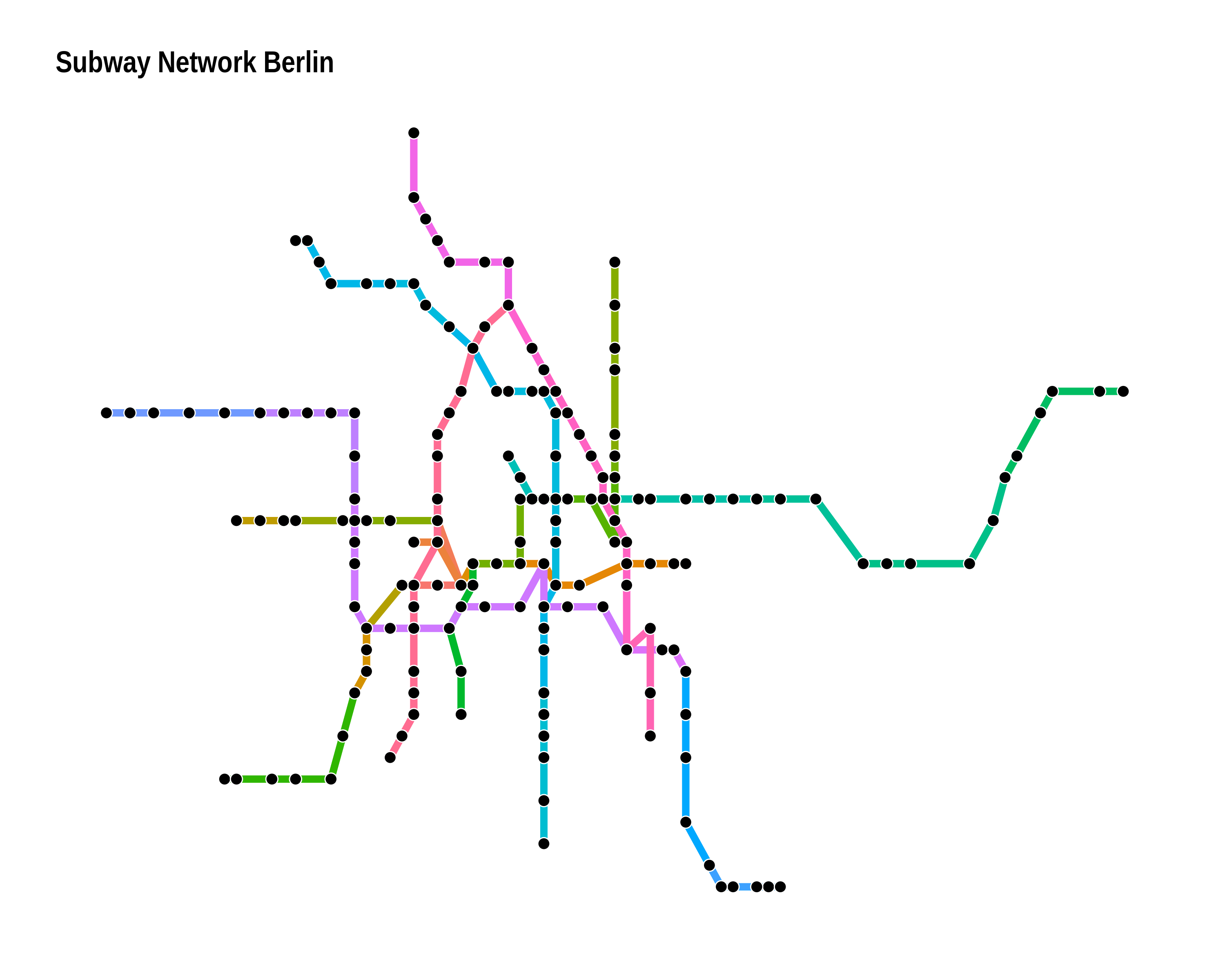An R package that implements several edge bundling/flow and metro map algorithms. So far it includes

• Force directed edge bundling
• Stub bundling (paper)
• Hammer bundling (python code)
• Edge-path bundling (paper)
• TNSS flow map (paper)
• Multicriteria Metro map layout (paper)

(The API is not very opinionated yet and may change in future releases.)

## Installation

The package is available on CRAN.

install.packages("edgebundle")

The developer version can be installed with

# install.packages("remotes")
remotes::install_github("schochastics/edgebundle")

Note that edgebundle imports reticulate and uses a pretty big python library (datashader).

library(edgebundle)
library(igraph)

## Edge bundling

The expected input of each edge bundling function is a graph (igraph/network or tbl_graph object) and a node layout.
All functions return a data frame of points along the edges of the network that can be plotted with {{ggplot2}} using geom_path() or geom_bezier() for edge_bundle_stub().

library(igraph)
g <- graph_from_edgelist(
matrix(c(1,12,2,11,3,10,4,9,5,8,6,7),ncol=2,byrow = T),F)
xy <- cbind(c(rep(0,6),rep(1,6)),c(1:6,1:6))

fbundle <- edge_bundle_force(g,xy,compatibility_threshold = 0.1)
#>            x       y     index group
#> 1 0.00000000 1.00000 0.0000000     1
#> 2 0.00611816 1.19977 0.0303030     1
#> 3 0.00987237 1.29767 0.0606061     1
#> 4 0.01929293 1.52427 0.0909091     1
#> 5 0.02790686 1.68643 0.1212121     1
#> 6 0.03440142 1.81285 0.1515152     1

The result can be visualized as follows.

library(ggplot2)

ggplot(fbundle) +
geom_path(aes(x, y, group = group, col = as.factor(group)),
size = 2, show.legend = FALSE) +
geom_point(data = as.data.frame(xy), aes(V1, V2), size = 5) +
theme_void()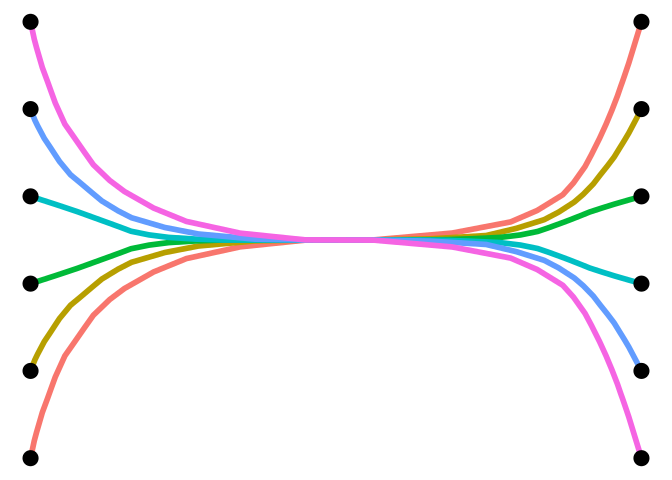# simple edge-path bundling example
g <- graph_from_edgelist(matrix(c(1, 2, 1, 6, 1, 4, 2, 3, 3, 4, 4, 5, 5, 6),
ncol = 2, byrow = TRUE), FALSE)
xy <- cbind(c(0, 10, 25, 40, 50, 50), c(0, 15, 25, 15, 0, -10))
res <- edge_bundle_path(g, xy, max_distortion = 2, weight_fac = 2, segments = 50)

ggplot() +
geom_path(data = res, aes(x, y, group = group, col = as.factor(group)),
size = 2, show.legend = FALSE) +
geom_point(data = as.data.frame(xy), aes(V1, V2), size = 5) +
scale_color_manual(values = c("grey66", "firebrick3", "firebrick3", rep("grey66", 4))) +
theme_void()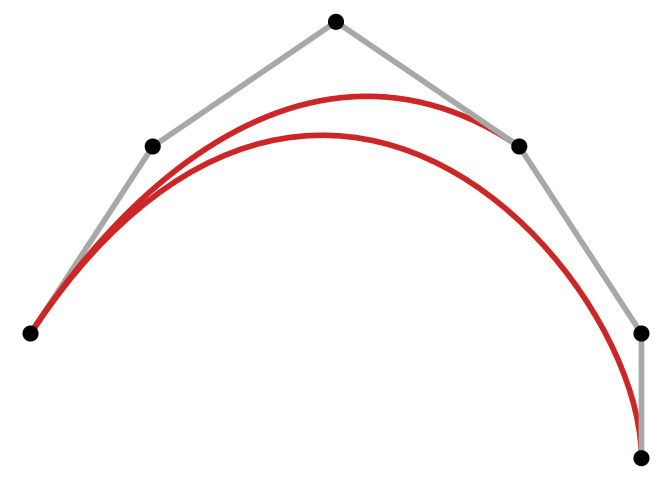For edge_bundle_stub(), you need geom_bezier() from the package {{ggforce}}.

library(ggforce)
g <- graph.star(10, "undirected")

xy <- matrix(c(
0, 0,
cos(90 * pi / 180), sin(90 * pi / 180),
cos(80 * pi / 180), sin(80 * pi / 180),
cos(70 * pi / 180), sin(70 * pi / 180),
cos(330 * pi / 180), sin(330 * pi / 180),
cos(320 * pi / 180), sin(320 * pi / 180),
cos(310 * pi / 180), sin(310 * pi / 180),
cos(210 * pi / 180), sin(210 * pi / 180),
cos(200 * pi / 180), sin(200 * pi / 180),
cos(190 * pi / 180), sin(190 * pi / 180)
), ncol = 2, byrow = TRUE)

sbundle <- edge_bundle_stub(g, xy, beta = 90)

ggplot(sbundle) +
geom_bezier(aes(x, y, group = group), size = 1.5, col = "grey66") +
geom_point(data = as.data.frame(xy), aes(V1, V2), size = 5) +
theme_void()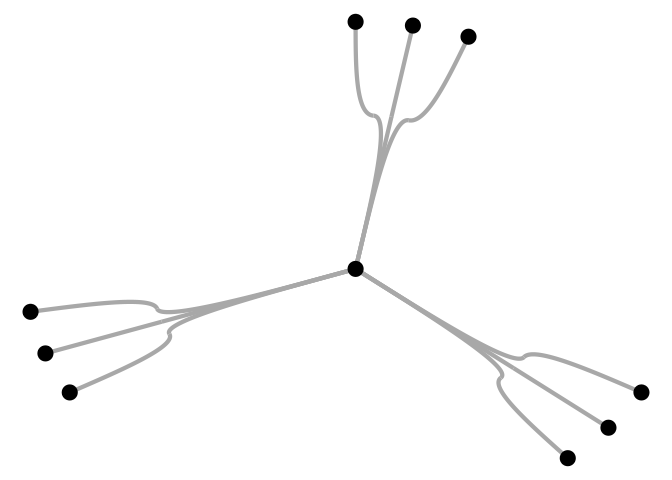The typical edge bundling benchmark uses a dataset on us flights, which is included in the package.

g <- us_flights
xy <- cbind(V(g)$longitude, V(g)$latitude)
verts <- data.frame(x = V(g)$longitude, y = V(g)$latitude)

fbundle <- edge_bundle_force(g, xy, compatibility_threshold = 0.6)
sbundle <- edge_bundle_stub(g, xy)
hbundle <- edge_bundle_hammer(g, xy, bw = 0.7, decay = 0.5)
pbundle <- edge_bundle_path(g, xy, max_distortion = 12, weight_fac = 2, segments = 50)

states <- map_data("state")

p1 <- ggplot() +
geom_polygon(data = states, aes(long, lat, group = group),
col = "white", size = 0.1, fill = NA) +
geom_path(data = fbundle, aes(x, y, group = group),
col = "#9d0191", size = 0.05) +
geom_path(data = fbundle, aes(x, y, group = group),
col = "white", size = 0.005) +
geom_point(data = verts, aes(x, y),
col = "#9d0191", size = 0.25) +
geom_point(data = verts, aes(x, y),
col = "white", size = 0.25, alpha = 0.5) +
geom_point(data = verts[verts$name != "", ], aes(x, y), col = "white", size = 3, alpha = 1) + labs(title = "Force Directed Edge Bundling") + ggraph::theme_graph(background = "black") + theme(plot.title = element_text(color = "white")) p2 <- ggplot() + geom_polygon(data = states, aes(long, lat, group = group), col = "white", size = 0.1, fill = NA) + geom_path(data = hbundle, aes(x, y, group = group), col = "#9d0191", size = 0.05) + geom_path(data = hbundle, aes(x, y, group = group), col = "white", size = 0.005) + geom_point(data = verts, aes(x, y), col = "#9d0191", size = 0.25) + geom_point(data = verts, aes(x, y), col = "white", size = 0.25, alpha = 0.5) + geom_point(data = verts[verts$name != "", ], aes(x, y),
col = "white", size = 3, alpha = 1) +
labs(title = "Hammer Edge Bundling") +
ggraph::theme_graph(background = "black") +
theme(plot.title = element_text(color = "white"))

alpha_fct <- function(x, b = 0.01, p = 5, n = 20) {
(1 - b) * (2 / (n - 1))^p * abs(x - (n - 1) / 2)^p + b
}

p3 <- ggplot() +
geom_polygon(data = states, aes(long, lat, group = group),
col = "white", size = 0.1, fill = NA) +
ggforce::geom_bezier(
data = sbundle, aes(x, y,
group = group,
alpha = alpha_fct(..index.. * 20)
), n = 20,
col = "#9d0191", size = 0.1, show.legend = FALSE
) +
ggforce::geom_bezier(
data = sbundle, aes(x, y,
group = group,
alpha = alpha_fct(..index.. * 20)
), n = 20,
col = "white", size = 0.01, show.legend = FALSE
) +
geom_point(data = verts, aes(x, y),
col = "#9d0191", size = 0.25) +
geom_point(data = verts, aes(x, y),
col = "white", size = 0.25, alpha = 0.5) +
geom_point(data = verts[verts$name != "", ], aes(x, y), col = "white", size = 3, alpha = 1) + labs(title = "Stub Edge Bundling") + ggraph::theme_graph(background = "black") + theme(plot.title = element_text(color = "white")) p4 <- ggplot() + geom_polygon(data = states, aes(long, lat, group = group), col = "white", size = 0.1, fill = NA) + geom_path(data = pbundle, aes(x, y, group = group), col = "#9d0191", size = 0.05) + geom_path(data = pbundle, aes(x, y, group = group), col = "white", size = 0.005) + geom_point(data = verts, aes(x, y), col = "#9d0191", size = 0.25) + geom_point(data = verts, aes(x, y), col = "white", size = 0.25, alpha = 0.5) + geom_point(data = verts[verts$name != "", ], aes(x, y),
col = "white", size = 3, alpha = 1) +
labs(title = "Edge-Path Bundling") +
ggraph::theme_graph(background = "black") +
theme(plot.title = element_text(color = "white"))

p1
p2
p3
p4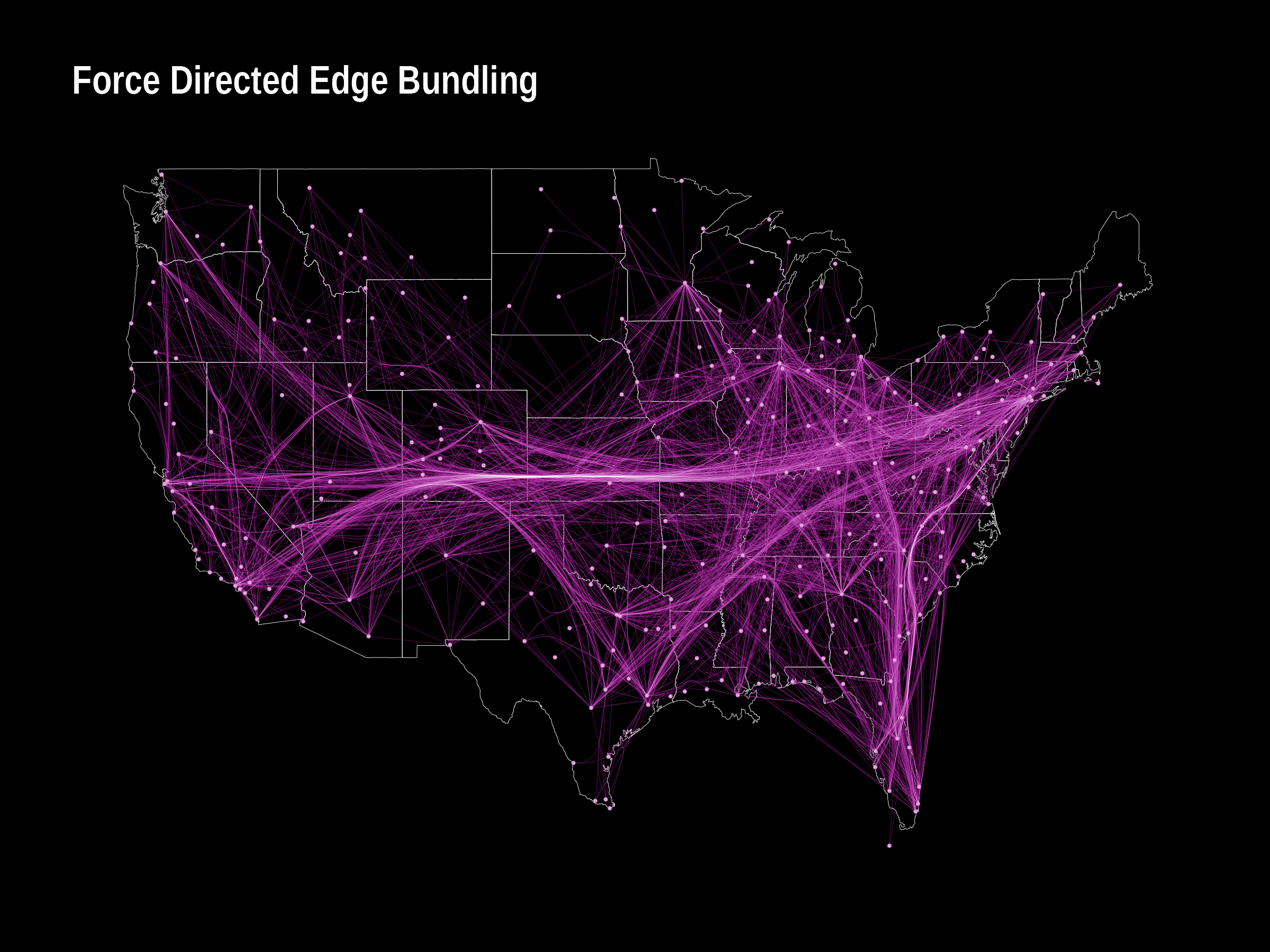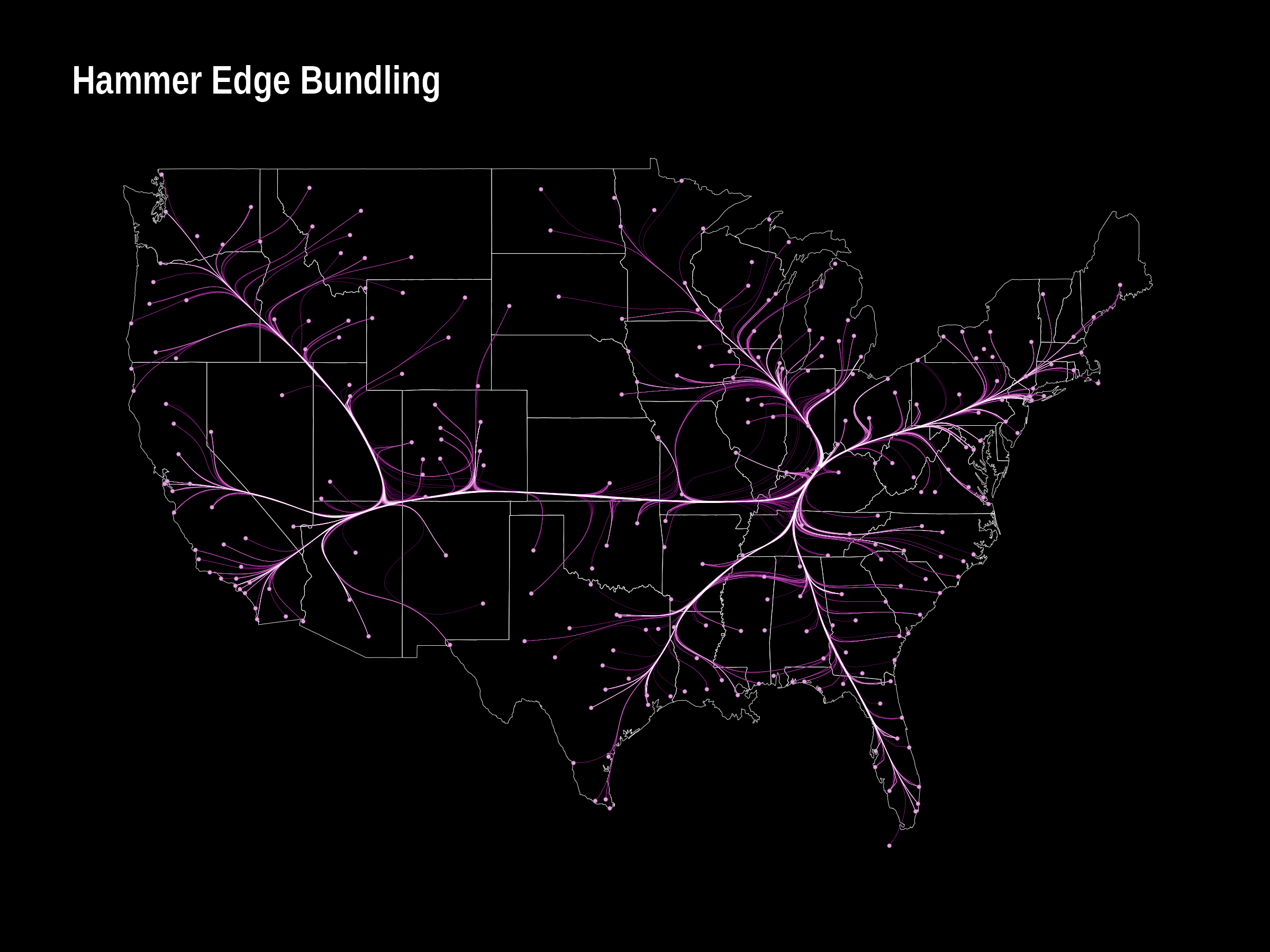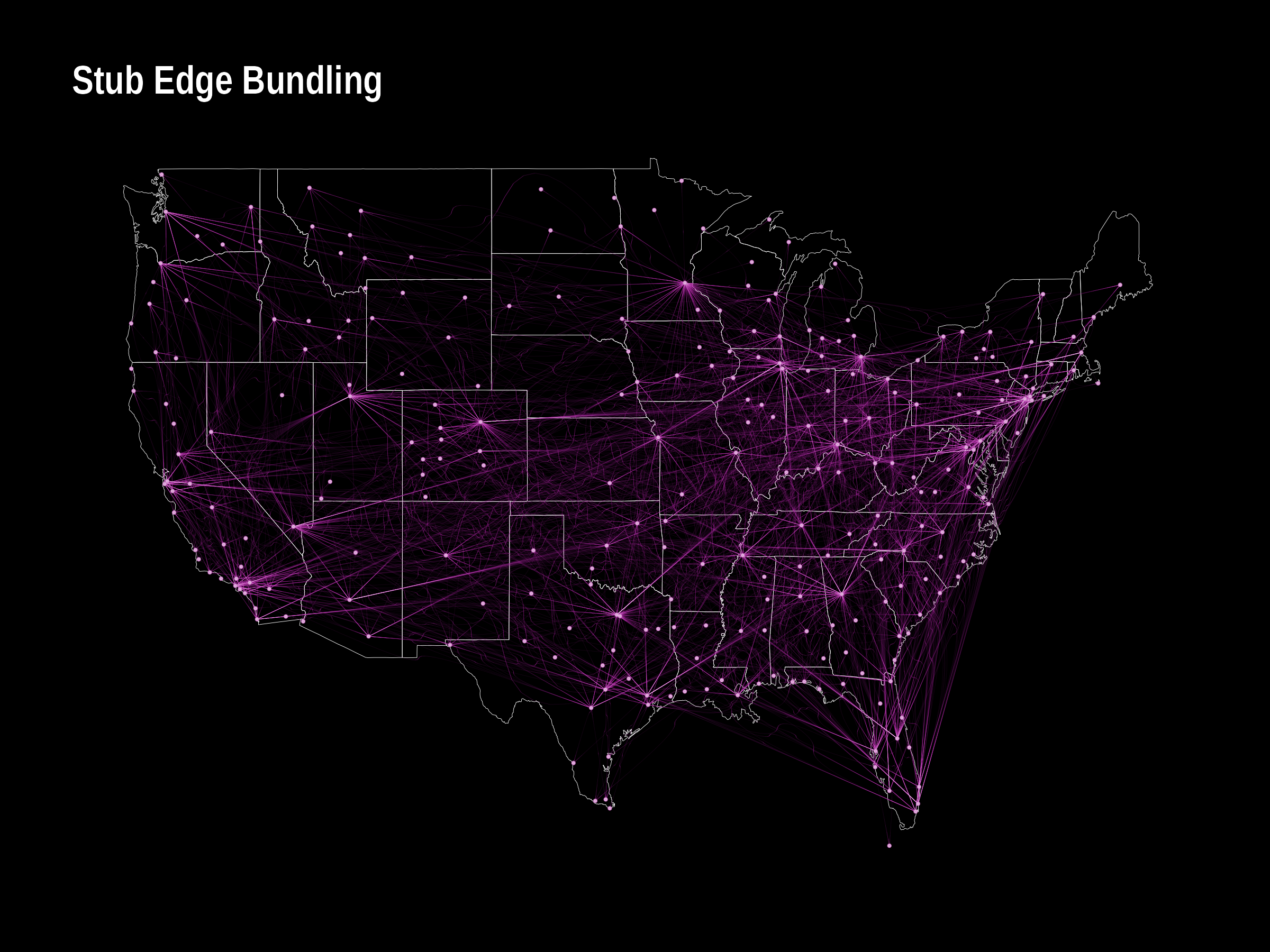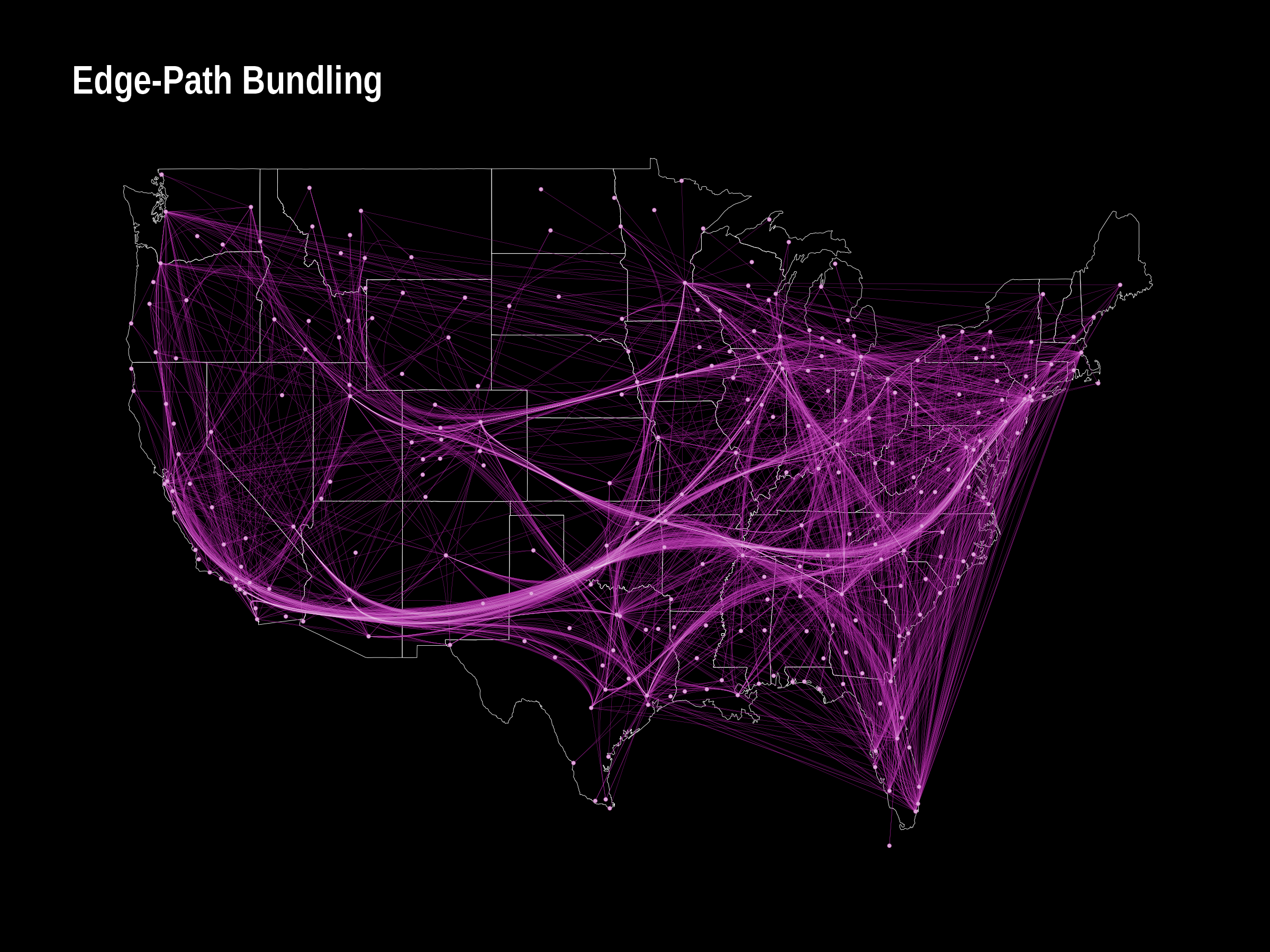## Flow maps

A flow map is a type of thematic map that represent movements. It may thus be considered a hybrid of a map and a flow diagram. The package so far implements a spatial one-to-many flow layout algorithm using triangulation and approximate Steiner trees.

The function tnss_tree() expects a one-to-many flow network (i.e. a weighted star graph), a layout for the nodes and a set of dummy nodes created with tnss_dummies().

library(ggraph)
xy <- cbind(state.center$x,state.center$y)[!state.name%in%c("Alaska","Hawaii"),]
xy_dummy <- tnss_dummies(xy,4)
gtree <- tnss_tree(cali2010,xy,xy_dummy,4,gamma = 0.9)

ggraph(gtree,"manual",x=V(gtree)$x,y=V(gtree)$y)+
geom_polygon(data=us,aes(long,lat,group=group),fill="#FDF8C7",col="black")+
lineend = "round",show.legend = FALSE)+
scale_edge_width(range=c(0.5,4),trans="sqrt")+
geom_node_point(aes(filter=tnss=="leaf"),size=1)+
geom_node_point(aes(filter=(name=="California")),size=5,shape=22,fill="#cc0000")+
theme_graph()+
labs(title="Migration from California (2010) - Flow map")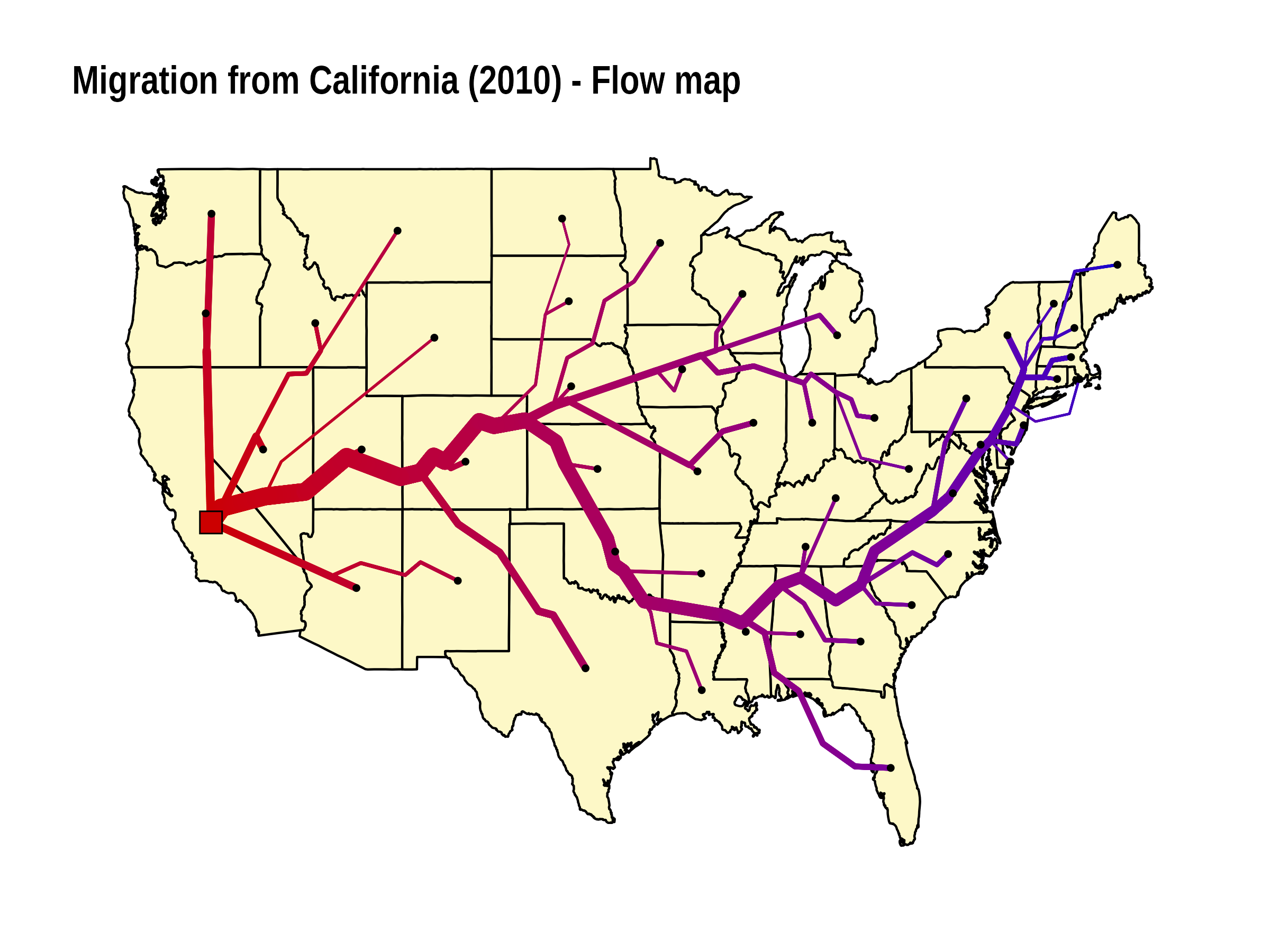To smooth the tree, use tnss_smooth(). Note that this changes the object type and you need to visualize it with {{ggplot2}} rather than {{ggraph}}.

smooth_df <- tnss_smooth(gtree,bw=5,n=20)

ggplot()+
geom_polygon(data=us,aes(long,lat,group=group),fill="#FDF8C7",col="black")+
geom_path(data = smooth_df,aes(x,y,group=destination,size=flow),
lineend = "round",col="firebrick3",alpha=1)+
theme_void()+
scale_size(range=c(0.5,3),guide = "none")+
labs(title="Migration from California (2010) - Flow map smoothed")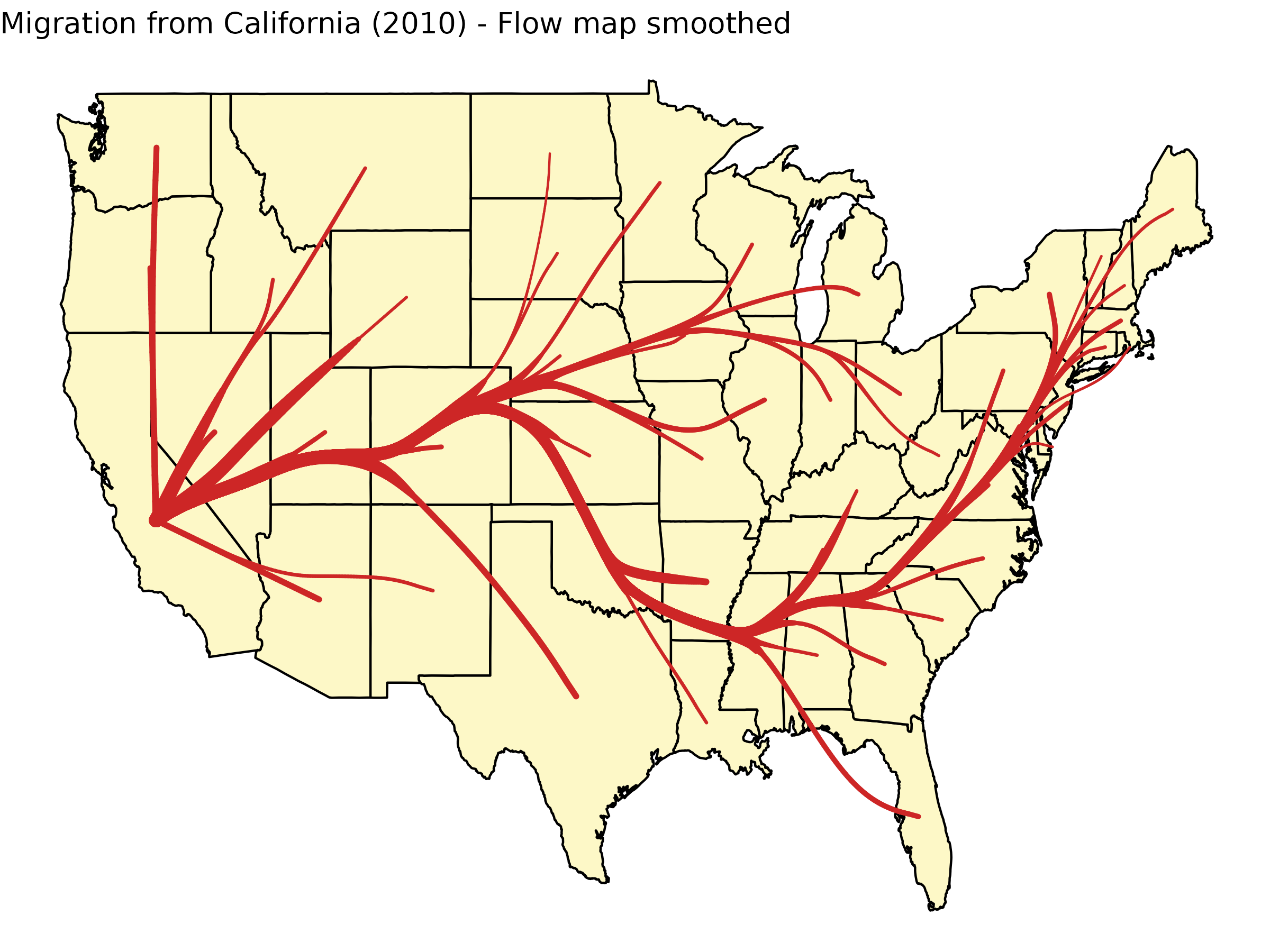See this gallery for more examples and code.

## Metro Maps

Metro map(-like) graph drawing follow certain rules, such as octilinear edges. The algorithm implemented in the packages uses hill-climbing to optimize several features desired in a metro map. The package includes the metro map of Berlin as an example.


# the algorithm has problems with parallel edges
g <- simplify(metro_berlin)
xy <- cbind(V(g)$lon,V(g)$lat)*100

# the algorithm is not very stable. try playing with the parameters
xy_new <- metro_multicriteria(g,xy,l = 2,gr = 0.5,w = c(100,100,1,1,100),bsize = 35)

# geographic layout
ggraph(metro_berlin,"manual",x=xy[,1],y=xy[,2])+
geom_node_point(shape=21,col="white",fill="black",size=3,stroke=0.5)

#schematic layout
ggraph(metro_berlin,"manual",x=xy_new[,1],y=xy_new[,2])+
labs(title = "Subway Network Berlin")